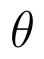Select Page

# 12 Science CBSE Maths Answers for MCQ Three Dimensional Geometry in English

12 Science CBSE Maths Answers for MCQ Three Dimensional Geometry in English to enable students to get Answers in a narrative video format for the specific question.

Expert Teacher provides 12 Science CBSE Maths Answers for MCQ Three Dimensional Geometry through Video Answers in English language. This video solution will be useful for students to understand how to write an answer in exam in order to score more marks. This teacher uses a narrative style for a question from Three Dimensional Geometry not only to explain the proper method of answering question, but deriving right answer too.

Please find the question below and view the Answer in a narrative video format.

Question:

## Similar Questions from CBSE, 12th Science, Maths, Three Dimensional Geometry

Question 1 : Find the vector equation of the plane which contains the line of intersection of the planesandand which is perpendicular to the plane(View Answer Video)

Question 2 : If a line marks anglesandwith x, y and z-axis respectively, whereis acute, then find. (View Answer Video)

Question 3 : A line passes through the point with position vectorand makes anglesandwith x, y and z-axis respectively. Find the equation of the line in the Cartesian form. (View Answer Video)

Question 4 : If a line has the direction ratios -18, 12, -4, then what are its direction cosines? (View Answer Video)

Question 5 : Write the sum of intercepts cut off by the planeon the three axes.  (View Answer Video)

### Probability

Question 1 :  A die is rolled. If E= (1, 3, 5), F(2, 3) and G= {2, 3, 4, 5}, find(View Answer Video)

Question 2 : An urn contains 4 balls. Two balls are drawn at random from the urn (without replacement) and are found to be white. What is the probability that all the four balls in the urn are white?  (View Answer Video)

Question 3 : One card is drawn from a pack of 52 cards. Find the probability of getting a king   (View Answer Video)

Question 4 : Consider the experiment of tossing a coin. If the coin shows head, toss is done again, but if it shows tail, then throw a die. Find the conditional probability of the events that ' the die shows a number greater than 4', given that ' there is atleast one tail'.    (View Answer Video)

Question 5 : One card is drawn from a pack of 52 cards. Find the probability of getting a red card:

### Relations and Functions

Question 1 : Functionsare defined respectively, by, find. (View Answer Video)

Question 2 : Consider a binary operation * on N defined as. Choose the correct answer. (View Answer Video)

Question 3 : Letdefined as f(x) = x be an identity function. Then, (View Answer Video)

Question 4 : Letbe defined as f(x) = 3x. Choose the correct answer. (View Answer Video)

Question 5 : Let * be the binary operation on N given by a * b = LCM of a and b. Find the identity of * in N? (View Answer Video)

### Determinant

Question 1 : Find the inverse of the matrix. (View Answer Video)

Question 2 : If, then x is equal to, (View Answer Video)

Question 3 : Find the values of x, if. (View Answer Video)

Question 4 : Find the value of k, if the area of the triangle is 4 sq unit and vertices are (k, 0) (4, 0) (0, 2). (View Answer Video)